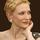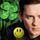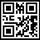## General Question# Can someone help me with my math assignment?

Asked by eishal (4) 1 week ago

The question is: You’re the representative on the student council and you’ve been assigned the task of finding a location to host a dance. You’ve narrowed it down to 3 options: Ancient abyss charges a \$200 flat fee and \$4/person. Beautiful Babylon charges a \$99 flat fee and \$6/person. Crazy Cleopatra charges a \$280 flat fee and \$3/person. Use substitution, elimination, or graphing to solve. Write a proposal outlining your recommendations.

Observing members: 0Composing members: 0We don’t do people’s homework here, but we can help in other ways. For instance, some of us can suggest an approach. One would hope, though, that your instructor has already prepared you to solve word problems like this one.

Jeruba (51639)“Great Answer” (5) Flag as…I am assuming that the problem is asking to find the least expensive choice. Without knowing the number of students, there is no way of deciding. Comparing any two of the options, the one with the higher flat fee has a lower per student fee.

What I would do is to graph the three choices as a function of number of students. You will get three lines. For 0 students, the cost will be in order of flat fee. As the number of students increases, the lines will intersect and the lowest cost will change. Eventually, the graphs will be in order of cost per student. For a report, I would track which option is least expensive for what range of students. The \$99 one will be cheapest for 0 to some number where the line with the \$99 dollar flat fee intersects one of the other lines. You could even use a different color to indicate which part of each line is the least expensive.

LostInParadise (28559)“Great Answer” (8) Flag as…Can you use Excel?
Make 4 columns
The first will be n, the number of students from 1 to 100. Then next 3 columns will be the cost of A, B and C for that number of students. For example : A is .200 + n*4
Then use autofill to fill in the amounts for 1 to 100 students.
You can auto-plot the results or look at the table of numbers . Obviously B is cheapest when there are few students. And C is cheapest when there are many students. A is somewhere in the middle. The graph will show you the crossover points.

LuckyGuy (39221)“Great Answer” (5) Flag as…There is missing data here, and without it you can’t give a perfect answer.

How many people are you expecting to attend? If you have that number, then the solution is simple math.

Since you don’t have that, you will need to give ranges instead:

If 20 people attend, then option 1 is <some number>, option 2 is <another number> and so on.

If 50 people attend, then…...

I think that’s what the teacher is really asking you to do.

elbanditoroso (29708)“Great Answer” (7) Flag as…Still need help?
Which one is the chepest if you have only 1 person. The answer is obvious. B.
Which is the cheapest if you have a lot of people. The answer is obvious C

Now let’s see where the crossover points are.
Find the point where A and B are equal. A is 200 + 4n B is 99 + 6n.
Set A = to B and solve for n 200 + 4 n = 99 + 6n Below that number B is cheaper. Above that A is cheaper until….
Lets see where A and C cross.
200 + 4n = 280 + 3n Solve for n. At that number of students, A and C are equal. Above that number C is cheaper.

LuckyGuy (39221)“Great Answer” (2) Flag as…As an aside, this seemingly elementary problem has some interesting possibilities. It is not the case in this particular problem (I graphed the lines with Geogebra), but the numbers could have been arranged so that the business in the middle is never the least expensive – it would always be more expensive than one of the others – not a very good business model.

LostInParadise (28559)“Great Answer” (0) Flag as…or